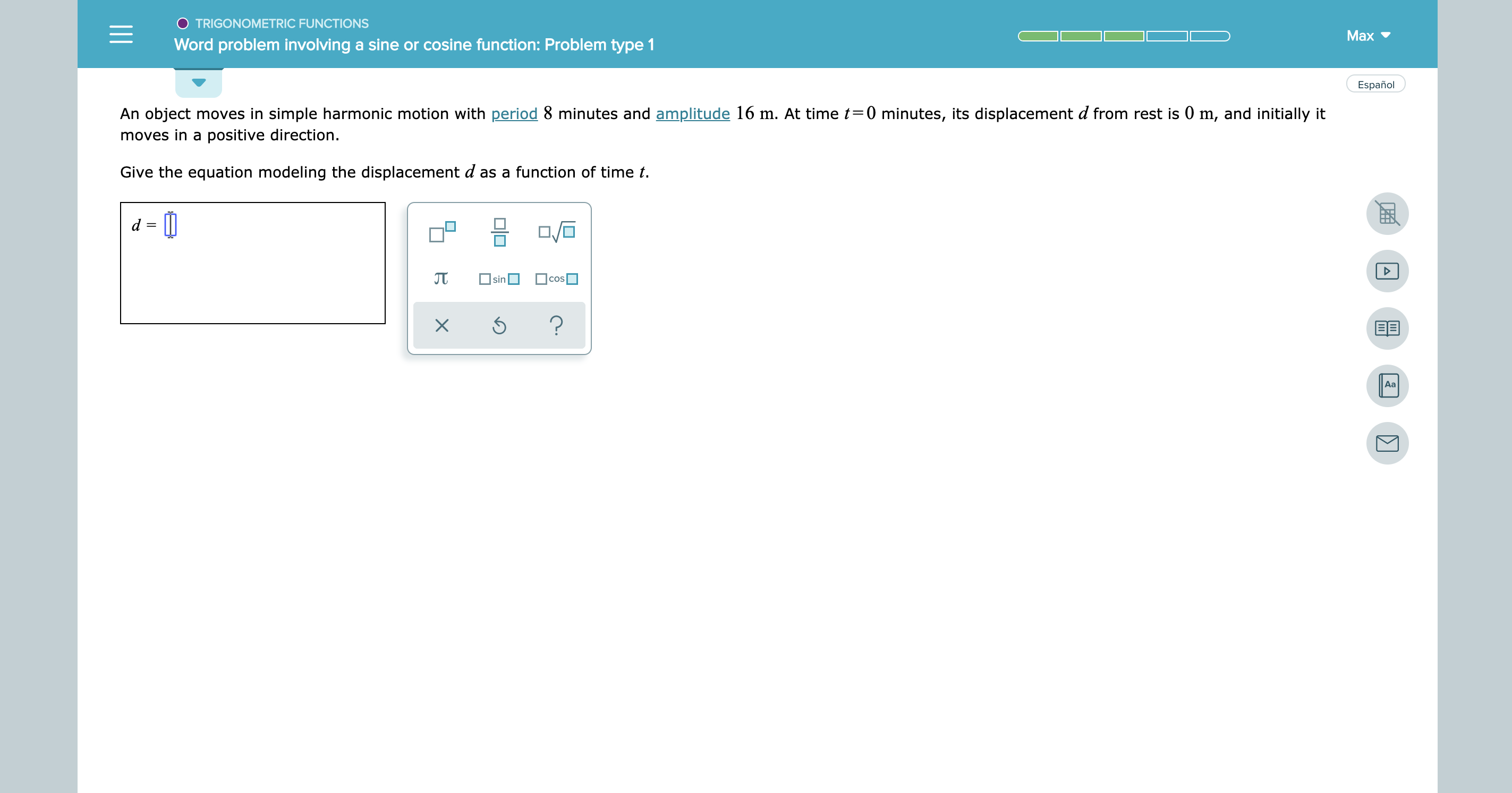# O TRIGONOMETRIC FUNCTIONSMaxWord problem involving a sine or cosine function: Problem type 1EspañolAn object moves in simple harmonic motion with period 8 minutes and amplitude 16 m. At time t 0 minutes, its displacement d from rest is 0 m, and initially itmoves in a positive direction.Give the equation modeling the displacement d as a function of time tsinCOS?EEAa

Question
3 views

See attachedhelp_outlineImage TranscriptioncloseO TRIGONOMETRIC FUNCTIONS Max Word problem involving a sine or cosine function: Problem type 1 Español An object moves in simple harmonic motion with period 8 minutes and amplitude 16 m. At time t 0 minutes, its displacement d from rest is 0 m, and initially it moves in a positive direction. Give the equation modeling the displacement d as a function of time t sin COS ? EE Aa fullscreen
check_circle

Step 1

Equation  modeling the diplacement d as function of time = A sin wt where A = amplitude and time period = 2π/w

Step 2

A = amplitude = 16 m

Period = 8 minutes = 8 x 60 = 480 second...

### Want to see the full answer?

See Solution

#### Want to see this answer and more?

Solutions are written by subject experts who are available 24/7. Questions are typically answered within 1 hour.*

See Solution
*Response times may vary by subject and question.
Tagged in

### Functions